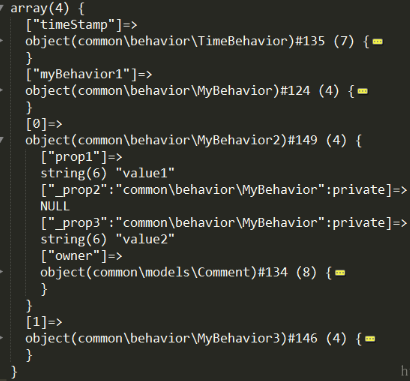## Yii2基本概念之——行为(Behavior)

Yii的行为就是这样一类对象，当一个对象(继承了Component的)想要扩展功能，又不想改变原有代码时，那么你完全可以用行为去实现这些新功能，然后绑定到该对象上——完全是符合“开闭原则”的。 Yii的行为都需要继承自yii\base\Behavior，而能接受行为绑定从而扩充自身功能的只能是yii\base\Component的子类，只继承BaseObject基类没有继承Component的不能享受此“待遇”。因此，行为是组件才有的功能。行为和事件结合起来使用，还可以定义组件在何种事件进行何种反馈。

1. 将属性和方法注入到一个component里面，被访问时和别的属性或者方法访问无异（行为的附加
2. 响应component中触发的事件，以便对某一事件作出反应（行为的绑定(事件)和触发，是对事件的应用

# 行为的附加

#### 1. 静态附加

class User extends ActiveRecord
{
const MY_EVENT = 'my_event';
public function behaviors()
{
return [

// 匿名行为，只有行为类名
MyBehavior::className(),

// 命名行为，只有行为类名
'myBehavior2' => MyBehavior::className(),

// 匿名行为，配置数组
[
'class' => MyBehavior::className(),
'prop1' => 'value1',
'prop2' => 'value2',
],

// 命名行为，配置数组
'myBehavior4' => [
'class' => MyBehavior::className(),
'prop1' => 'value1',
'prop2' => 'value2',
]
];
}
}

as 前缀方法.

[
'class' => User::className(),
'as myBehavior2' => MyBehavior::className(),
'as myBehavior3' => [
'class' => MyBehavior::className(),
'prop1' => 'value1',
'prop3' => 'value3',
],
]

#### 2. 动态附加

use app\components\MyBehavior;
// 附加行为——对象
$user->attachBehavior('myBehavior1', new MyBehavior); // 附加行为——类名$user->attachBehavior('myBehavior2', MyBehavior::className());
// 附加行为——配置数组
$user->attachBehavior('myBehavior3', [ 'class' => MyBehavior::className(), 'prop1' => 'value1', 'prop2' => 'value2', ]); 也可以通过 yii\base\Component::attachBehaviors()同时附加多个行为： $myBehavior = new MyBehavior();
$user->attachBehaviors([ 'myBehavior1'=>$myBehavior,
[
'class' => MyBehavior2::className(),
'prop1' => 'value1',
'prop3' => 'value2',
],
new MyBehavior3()
]);

private $_behaviors;$_behaviors的数据结构：Component::behaviors()
Component::ensureBehaviors()
Component::attachBehaviorInternal()
Behavior::attach()

Component::behaviors()用于供子类覆写(方法重写)，比如：

public function behaviors()
{
return [
'timeStamp' => [
'class' => TimeBehavior::className(),
'create' => 'create_at',
'update' => 'update_at',
],
];
}

yii\base\Component::ensureBehaviors()方法经常出现，它的作用是将各种动态的和静态的方式附加的行为变成标准格式(参看$_behaviors的数据结构)： public function ensureBehaviors() { if ($this->_behaviors === null) {
$this->_behaviors = []; // behaviors()方法由Component的子类重写 foreach ($this->behaviors() as $name =>$behavior) {
$this->attachBehaviorInternal($name, $behavior); } } } 我们会发现 yii\base\Component 类中基本所有的方法(和属性或者方法相关的方法，为了属性注入)里面都调用了 ensureBehaviors() 此方法，为什么要这样做呢？目的就是为了确保该方法一定会被执行到。 这就是为什么我们在系统中没有单独提议的去执行调用 他（如在 init()方法去调用），但是最后 ensureBehaviors()一样被执行了，因为在系统在进行组件，或者其他初始化时，一定会调用到 yii\base\Component 类中的接触方法， 如 yii\base\Component ::__set(), yii\base\Component __get() ,等，因为这些在进行组件初始化动态赋值时，肯定会被调用到。 你想测试下系统什么时候最开始调用的 ensureBehaviors() 方法，你可以在该方法中输出trace 。。在方法中加入 print_r(\debug_trace()) 就可以看到 方法被调用了 既然 ensureBehaviors 肯定会被执行，就是说 只要我们重写子类的 behaviors（），只要有行为，肯定就会被绑定到类中 接下来的第三个出场的attachBehaviorInternal(),我们看看是何方神圣： private function attachBehaviorInternal($name, $behavior) { //如果是配置数组，那就将其创建出来再说 if (!($behavior instanceof Behavior)) {
$behavior = Yii::createObject($behavior);
}
if (is_int($name)) { // 匿名行为 //先是行为本身和component绑定$behavior->attach($this); //$this 的妙用，将自身和行为直接进行绑定，行为的owner 就是组件(Componet)对象 ，这样就将两者建立了关系，随处可调用。
//将行为放进$_behaviors数组，没有键值的是匿名行为$this->_behaviors[] = $behavior; } else { //命名行为 if (isset($this->_behaviors[$name])) { //命名行为需要保证唯一性$this->_behaviors[$name]->detach(); }$behavior->attach($this); //命名行为，键值就是行为名称$this->_behaviors[$name] =$behavior;
}

return $behavior; } Yii中以Internal开头或者结尾的，一般是私有方法，往往都是命门所在，如果要看源码，这些都是核心逻辑实现的地方。 最后一个出场的是Behavior::attach()，Behavior有一个属性$owner，指向是拥有它的组件，就是行为的拥有者。组件和行为是一个相互绑定、相互持有的过程。组件在$_behavior持有行为的同时，行为也在$owner中持有组件。

public function attach($owner) { //Behavior的$owner指向的是行为的所有者
$this->owner =$owner;
//让行为的所有者$owner绑定用户在Behavior::events()中所定义的事件和处理器 foreach ($this->events() as $event =>$handler) {
$owner->on($event, is_string($handler) ? [$this, $handler] :$handler);
}
}

#### 行为的解除

//解除所有行为
$user->detachBehaviors(); 这上面两种方法，都会调用到 yii\base\Behavior::detach() ，其代码如下: public function detachBehavior($name)
{
$this->ensureBehaviors(); if (isset($this->_behaviors[$name])) {$behavior = $this->_behaviors[$name];
//1.将行为从$owner的$_behaviors中删除
unset($this->_behaviors[$name]);
//2.解除$owner的所有事件和其处理器$behavior->detach();
return $behavior; } return null; } $behavior->detach()是这样的：

public function detach()
{
if ($this->owner) { //解绑$owner所有事件和其事件处理器
foreach ($this->events() as$event => $handler) {$this->owner->off($event, is_string($handler) ? [$this,$handler] : $handler); } //$owner重新置为null，表示没有任何拥有者
$this->owner = null; } } 在 Component 类中，我们看见 __clone 组件时，会将所有的 行为，事件都清空  public function __clone() {$this->_events = [];
$this->_eventWildcards = [];$this->_behaviors = null;
}

#### 行为所要响应的事件

class MyBehavior extends Behavior
{
public $attr; public function events() //覆写events方法 { return [ ActiveRecord::EVENT_BEFORE_INSERT => 'beforeInsert', //将事件和事件处理器绑定 User::MY_EVENT => [$object, 'methodName'],//自己定义的事件
];
}

//$event可以带来三个信息，事件名，触发此事件的对象（类或者实例），附加的数据 public function beforeInsert($event)
{
$model =$this->owner;//访问已附件的组件
// Use $model->attr } public function methodName($event)
{
$owner =$this->owner;//行为的拥有者
$sender =$event->sender//触发此事件的类或者实例
$data =$event->data;//触发事件时传递的参数

// Use $model->attr } }  events()方法返回一个关联数组，键是事件名，值是要响应的事件处理器。事件处理器可以是一下四种形式： • 此行为中的方法methodName，等效为[$this, 'methodName']
• 对象的方法：[$object, 'methodName'] • 类的静态方法：['Page', 'methodName'] • 闭包：function ($event) { ... }

1. 系统本身初始化，会调用类 Component::ensureBehaviors() 方法；

2.  Component::ensureBehaviors() 方法 ，会调用 $this->behaviors()，也就是我们在子类中重写的方法； 3. 调用ensureBehaviors() 方法，就会将规范好的行为对象，绑定到到 Component组件上(子类)，同时保存行为 到$this->_behaviors 的私有变量中；接着将行为中定义的event()事件 注册到组件上，保存到 $this->_events 的私有变量中。 4. 当我们在行为中定义的是系统中已有的 事件， 如 Model 中的 beforeSave, afterSave 等事件，该事件自然就会被触发了。 5. Component 类中的 __set(), __get(), __call() 方法中 都会通过$this->_behaviors 拿到行为对象，然后在行为对象中 找 变量，或者 方法 ，这样就达到了属性的注入了，所以就如我们说 Component子类 调用 行为的属性就像调用自己的属性一样。

## 行为响应事件的实例

Yii费了那么大劲，主要就是为了将行为中的事件handler绑定到类中去。因为在编程中用的最多的，也就是Component对各种事件的响应。通过行为注入，可以在不修改现有类的代码的情况下更改、扩展类对于事件的响应和支持。使用这个技巧，可以玩出很酷的花样出来。 比如，Yii自带的 yii\behaviors\AttributeBehavior 类，定义了在一个 ActiveRecord 对象的某些事件发生时， 自动对某些字段进行修改的行为。它有一个很常用的子类 yii\behaviors\TimeStampBehavior 用于将指定的字段设置为一个当前的时间戳。现在以它为例子说明行为的运用。 在 yii\behaviors\AttributeBehavior::event() 中，代码如下:

public function events()
{
return array_fill_keys(
array_keys($this->attributes), 'evaluateAttributes' ); }  代码很容易看懂，无需详述。 而在yii\behaviors\TimeStampBehavior::init()中有代码： public function init() { parent::init(); if (empty($this->attributes)) {
//重点看这里
$this->attributes = [ BaseActiveRecord::EVENT_BEFORE_INSERT => [$this->createdAtAttribute, $this->updatedAtAttribute], BaseActiveRecord::EVENT_BEFORE_UPDATE =>$this->updatedAtAttribute,
];
}
}


return [
BaseActiveRecord::EVENT_BEFORE_INSERT => 'evaluateAttributes',
BaseActiveRecord::EVENT_BEFORE_UPDATE => 'evaluateAttributes',
];


public function evaluateAttributes($event) { ... if (!empty($this->attributes[$event->name])) {$attributes = (array) $this->attributes[$event->name];
//这里默认返回默认的时间戳time()
$value =$this->getValue($event); foreach ($attributes as $attribute) { if (is_string($attribute)) {
if ($this->preserveNonEmptyValues && !empty($this->owner->$attribute)) { continue; } //将其赋值给$owner的字段
$this->owner->$attribute = $value; } } } }  使用时，只需要在ActiveRecord里面重载behaviors()方法： public function behaviors() { return [ [ 'class' => TimestampBehavior::className(), 'attributes' => [ ActiveRecord::EVENT_BEFORE_INSERT => 'created_at', ActiveRecord::EVENT_BEFORE_UPDATE => 'updated_at', ] ], ]; }  因此，当EVENT_BEFORE_INSERT事件触发， 这样，你在插入记录时created_atupdated_at会自动更新，而在修改时updated_at会更新。 #### 行为的属性和方法注入原理 通过以上各个例子，组件附加了行为之后，就获得了行为的属性和方法。那么，这是如何实现的呢？归根结底主要通过__set()，__get()，__call()这些魔术方法来实现的。属性的注入靠的是__set()，__get()，而方法的注入是靠__call() ###### 属性的注入 Component持有一个数组$_behavior，里面都是Behavior子类，而Behavior继承自Yii最基础的BaseObject。在《Yii2基本概念之——属性(property)》中我们介绍了属性的概念，因此Behavior也是可以运用属性的。

Component的可读属性，我们看看Component的getter函数：

public function __get($name) {$getter = 'get' . $name; //这是自己的可写属性 if (method_exists($this, $getter)) { return$this->$getter(); } /**下面是比BaseObject多出来的部分**/$this->ensureBehaviors();
//依次检查各个行为中的可读属性
foreach ($this->_behaviors as$behavior) {
if ($behavior->canGetProperty($name)) {
return $behavior->$name;
}
}
...
}


Component的可写属性，我们看看Component的setter函数：

public function __set($name,$value)
{
$setter = 'set' .$name;
//自己的可写属性
if (method_exists($this,$setter)) {
$this->$setter($value); return; } elseif (strncmp($name, 'on ', 3) === 0) {
$this->on(trim(substr($name, 3)), $value); return; } elseif (strncmp($name, 'as ', 3) === 0) {
$name = trim(substr($name, 3));
$this->attachBehavior($name, $value instanceof Behavior ?$value : Yii::createObject($value)); return; }$this->ensureBehaviors();
//依次检查各个行为中是否有可写属性$name foreach ($this->_behaviors as $behavior) { if ($behavior->canSetProperty($name)) {$behavior->$name =$value;
return;
}
}
...
}


• 自己的setter函数，也即是自己的可写属性
• 如果$name是'on xyz'形式，则xyz作为事件，$value作为handler，将其绑定
• 如果$name是'as xyz'形式，则xyz作为行为名字，$value作为行为，将其附加
• 依次检查各个行为中是否有可写属性$name，返回第一个；如果没有则抛出异常 因此，Component的可读属性就是本身的可读属性加上所有行为的可读属性；而可写属性就是本身的可写属性加上所有行为的可写属性。 ###### 方法的注入 同属性的注入类似，方法的注入也是自身的方法加上所有行为的方法： public function __call($name, $params) {$this->ensureBehaviors();
//遍历所有行为的方法
foreach ($this->_behaviors as$object) {
if ($object->hasMethod($name)) {
return call_user_func_array([$object,$name], \$params);
}
}
...


#### 结语

posted on 2019-05-21 15:59  追风的浪子  阅读(204)  评论(0编辑  收藏